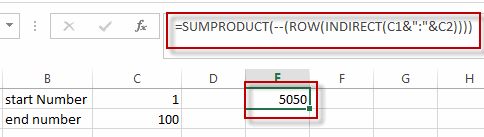## Sum Numbers from 1 to N

This post will guide you how to sum the numbers from 1 to N in Excel Worksheet. How do I sum the integers from 1 to N with an excel formula. How to write an excel formula to calculate 1+2+3+…+N in excel.

Normally, we can use the SUM function to sum a list of numbers easily. But if you supply a number N and you want to calculate the numbers from 1 to N . How to achieve it.

Assuming that you supply a number 100, and you want to sum the numbers from 1 to 100 (1+2+3+…+100), you need to create a formula based on the SUMPRODUCT function, the ROW function and the INDIRECT function to achieve the result. Like this:

`=SUMPRODUCT(--(ROW(INDIRECT(C1&":"&C2))))`

Type this formula into the formula box of a blank cell and press Enter key in your keyboard.You will see that the numbers from 1 to 100 has been added up. And you just need to update the end number in Cell C2 as you need to sum numbers.

### Related Functions

• Excel SUMPRODUCT function
The Excel SUMPRODUCT function multiplies corresponding components in the given one or more arrays or ranges, and returns the sum of those products.The syntax of the SUMPRODUCT function is as below:= SUMPRODUCT (array1,[array2],…)…
• Excel ROW function
The Excel ROW function returns the row number of a cell reference.The ROW function is a build-in function in Microsoft Excel and it is categorized as a Lookup and Reference Function.The syntax of the ROW function is as below:= ROW ([reference])….
• Excel INDIRECT function
The Excel INDIRECT function returns the cell reference based on a text string, such as: type the text string “A2” in B1 cell, it just a text string, so you can use INDIRECT function to convert text string as cell reference….